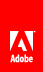# 绘制路径

Flash Player 10 和更高版本，Adobe AIR 1.5 和更高版本

 有关绘制线条和曲线的节中（请参阅绘制线条和曲线）介绍了一些命令，它们可用于绘制单个线条 (Graphics.lineTo()) 或曲线 (Graphics.curveTo())，然后将得到的线移至另一点 (Graphics.moveTo())，从而组成形状。Graphics.drawPath() 和 Graphics.drawTriangles() 方法接受一组对象，它们将那些相同的绘图命令表示为一个参数。使用这些方法，您可以提供一系列 Graphics.lineTo()、Graphics.curveTo()、Graphics.moveTo() 命令，使 Flash 运行时在一个单独的语句中执行这些命令。 GraphicsPathCommand 枚举类定义一组对应于绘图命令的常量。您可以将这一系列常量（包装在 Vector 实例中）作为对 Graphics.drawPath() 方法的一个参数来传递，然后通过一个单独的命令便可以呈现整个形状或多个形状。您还可以更改传递给这些方法的值，以更改现有形状。 除了这个绘图命令 Vector 之外，drawPath() 方法还需要一组坐标来对应每个绘图命令的坐标。创建一个包含坐标的 Vector 实例（Number 实例），将其作为第二个参数 (data) 传递给 drawPath() 方法。 注： 矢量中的值不是 Point 对象；该矢量是一系列数字，其中由两个数字组成的每个组表示一个 x/y 坐标对。 Graphics.drawPath() 方法将每个命令与其各自的点值（由两个或四个数字组成的集合）相匹配，以在 Graphics 对象中生成路径： ```package { import flash.display.*; public class DrawPathExample extends Sprite { public function DrawPathExample(){ var squareCommands:Vector. = new Vector.(5, true); squareCommands = GraphicsPathCommand.MOVE_TO; squareCommands = GraphicsPathCommand.LINE_TO; squareCommands = GraphicsPathCommand.LINE_TO; squareCommands = GraphicsPathCommand.LINE_TO; squareCommands = GraphicsPathCommand.LINE_TO; var squareCoord:Vector. = new Vector.(10, true); squareCoord = 20; //x squareCoord = 10; //y squareCoord = 50; squareCoord = 10; squareCoord = 50; squareCoord = 40; squareCoord = 20; squareCoord = 40; squareCoord = 20; squareCoord = 10; graphics.beginFill(0x442266);//set the color graphics.drawPath(squareCommands, squareCoord); } } }```Twitter™ 与 Facebook 中的内容不在 Creative Commons 的条款约束之下。